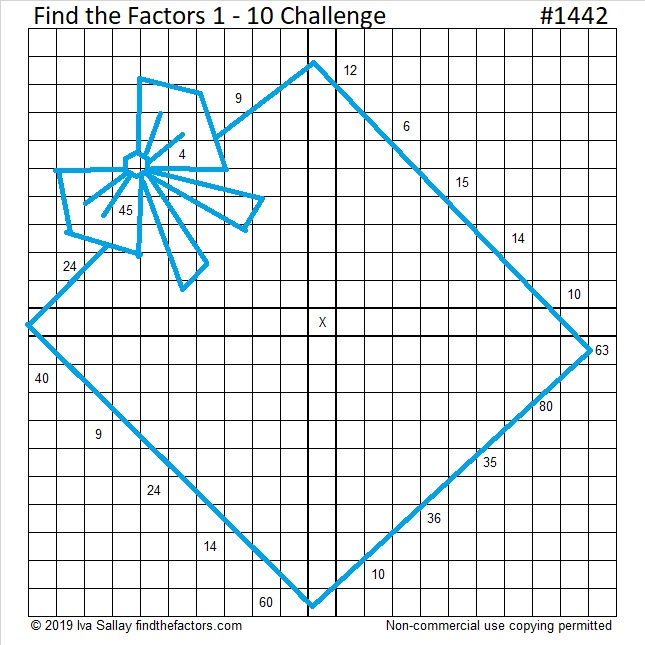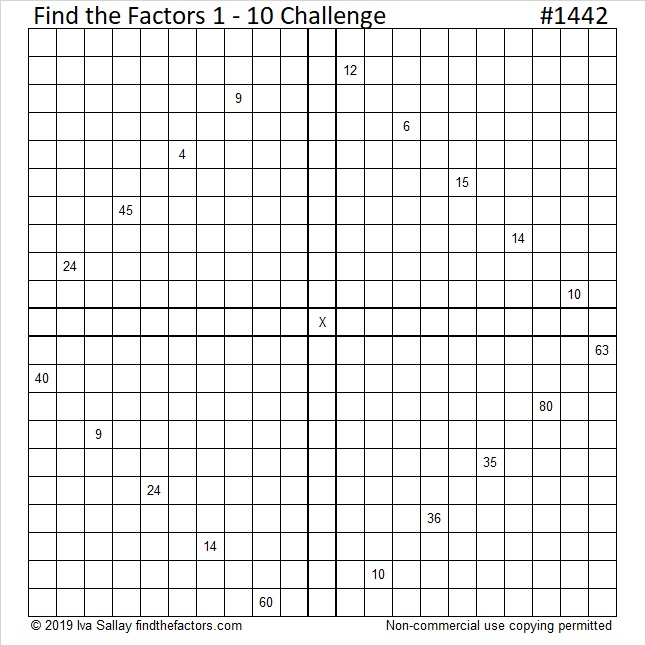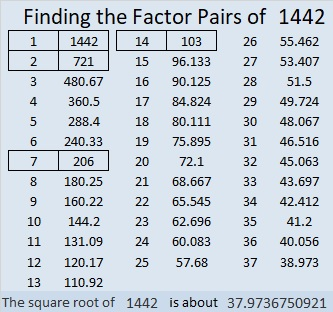# 1442 A Birthday Present for My Brother, Andy

My brother, Andy, has a birthday today. He’s very good at solving puzzles, so I made this Challenge puzzle as a  present for him.  Have a very happy birthday, Andy!You can try to solve it too. If the box and ribbon are too distracting, here’s a copy of the puzzle without the added color. Click on it to see it better.Print the puzzles or type the solution in this excel file: 10 Factors 1432-1442

That was puzzle number 1442. Here are some facts about that number:

• 1442 is a composite number.
• Prime factorization: 1442 = 2 × 7 × 103
• 1442 has no exponents greater than 1 in its prime factorization, so √1442 cannot be simplified.
• The exponents in the prime factorization are 1, 1, and 1. Adding one to each exponent and multiplying we get (1 + 1)(1 + 1)(1 + 1) = 2 × 2 × 2 = 8. Therefore 1442 has exactly 8 factors.
• The factors of 1442 are outlined with their factor pair partners in the graphic below.2(103)(7) = 1442, making it a leg in this Pythagorean triple:
1442-10560-10658 calculated from 2(103)(7), 103² – 7², 103² + 7²